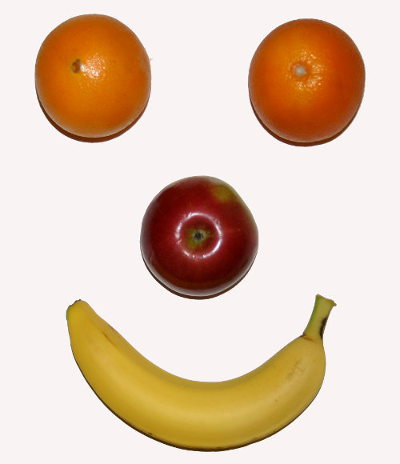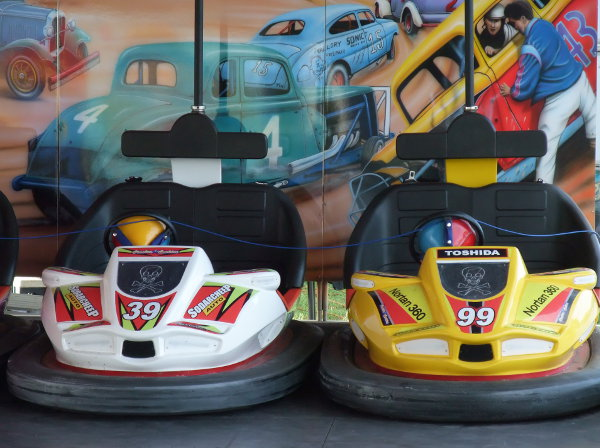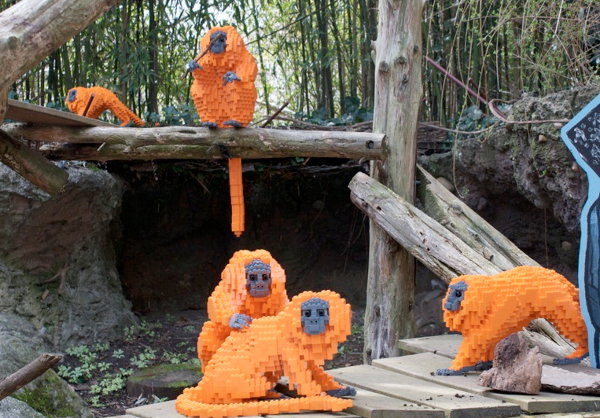# Distributive Law

## Example One - Fruit FaceThis fruit face has 2 oranges, 1 apple and 1 banana.

(a) Write this algebraically using variables such as a for apple, b for banana and o for orange.
(b) How many of each type of fruit would be in 10 fruit faces? Write this in algebra.

(a) 1a + 1b + 2o
(b) 10 × (1a + 1b + 2o) = 10a + 10b + 20o

## Example Two - Bumper CarsA bumper car manufacturer produces carnival sets, each with 5 white and 4 yellow bumper cars.

(a) Write this algebraically using variables such as w for a white car and y for a yellow car.
(b) How many of each colour would be in 3 carnival sets? Write this in algebra.

(a) 5w + 4y
(b) 3 × (5w + 4y) = 15w + 12y

## Questions

Expand these expressions:
Q1. (3a + 4b) × 5
Q2. (2x + 5y) × 3
Q3. 5 × (4p – 2q)

A1. 15a + 20b
A2. 6x + 15y
A3. 20p – 10q

## Factorizing Equations:

Factorizing is grouping terms by their greatest common factor. It is the reverse of expanding equations.

## Example Three - Rock ConcertEach band at a gig has 3 guitars and 1 drum kit.

(a) Write this algebraically using variables such as g for guitar and d for drum kit.
(b) A mix-up has occurred with the storage of music equipment. There are 15 guitars and 5 drum kits in a van. How many bands are playing? Write this in algebra.

(a) 3g + 1d
(b) 15g + 5d = 5 × (3g + 1d)
There are 5 bands playing.

## Example Four - Lego MonkeyEach Lego monkey uses 300 orange, 20 grey and 10 black blocks.

(a) Write this algebraically using variables such as b for black, g for grey and o for orange.
(b) How many Lego monkeys can be made from 1800 orange, 180 grey and 60 black blocks? Write this in algebra.

(a) 300 o + 20 g + 10 b
(b) 1800 o + 20 g + 10 b = 6 × (300 o + 20 g + 10 b)

## Questions

Factorize these expressions:
Q1. 20x + 15y
Q2. 14ab + 7a
Q3. 2xy – x